# Python 列表详解4

``````name = ['美杜莎', '古薰儿', '云韵', '雅妃']
print(name[0:2])``````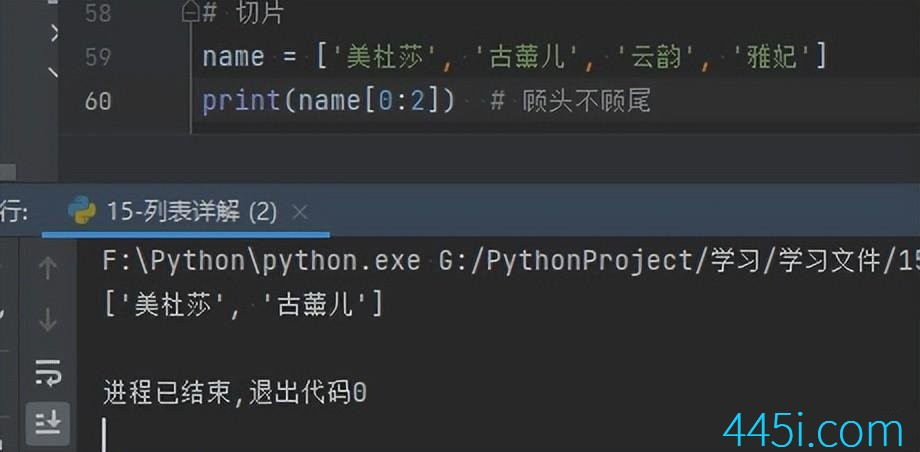``````name = ['美杜莎', '古薰儿', '云韵', '雅妃']
print(name[::2])``````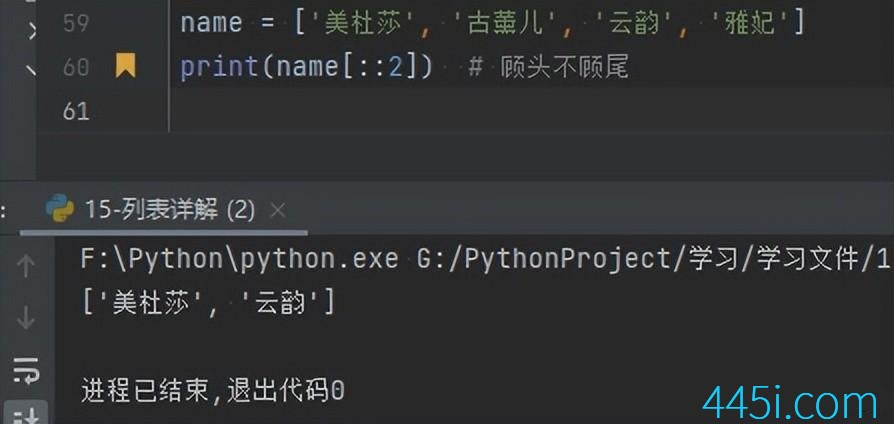``````name = ['美杜莎', '古薰儿', '云韵', '雅妃']
print(len(name))``````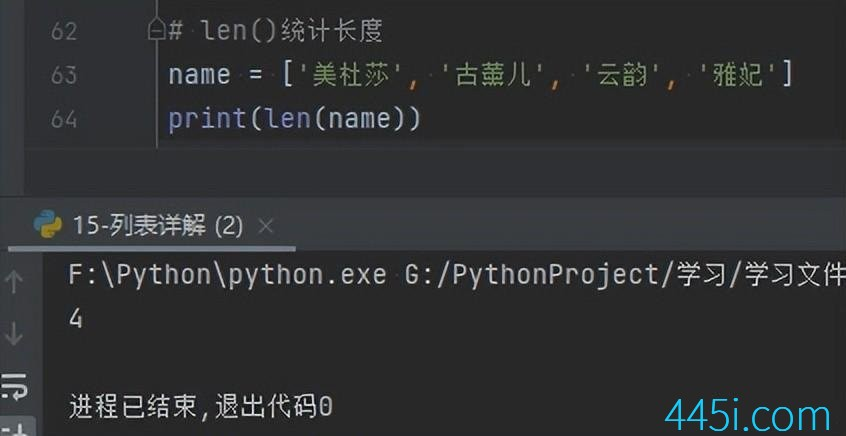``````name = ['美杜莎', '古薰儿', '云韵', '雅妃']
print('云韵' in name)````````````name = ['美杜莎', '古薰儿', '云韵', '雅妃']
for i in name:
print(i)``````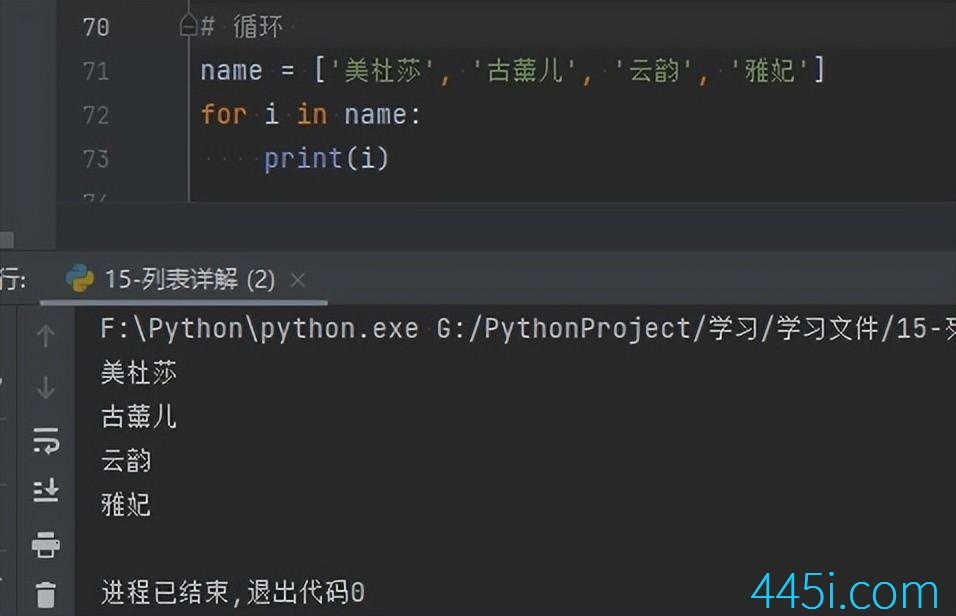``````name = ['美杜莎', '古薰儿', '云韵', '雅妃']
for i in name:
name.pop(0)
print(name)````````````name = ['美杜莎', '古薰儿', '云韵', '雅妃', '美杜莎', '美杜莎', '美杜莎']
print(name.count('美杜莎'))``````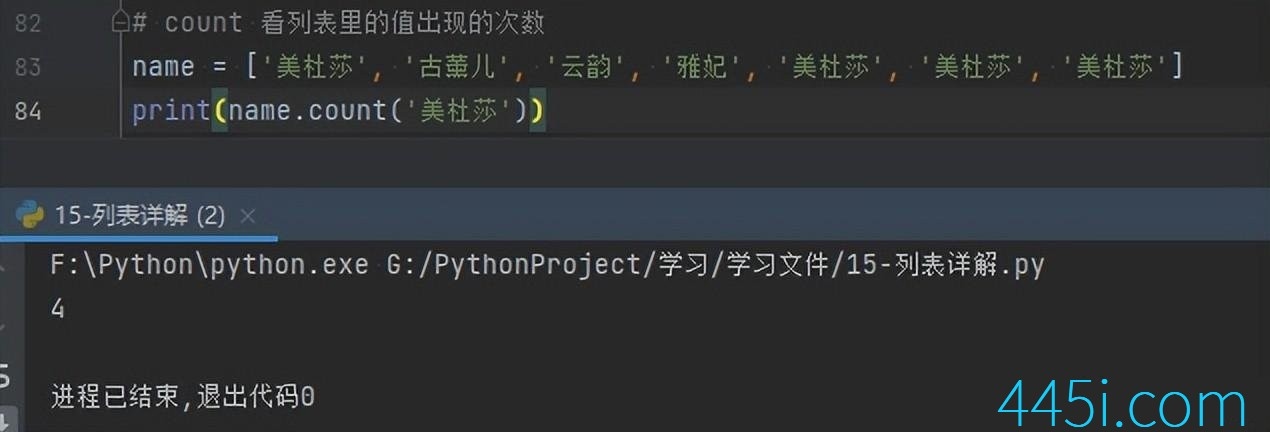``````name = ['美杜莎', '古薰儿', '云韵', '雅妃', '美杜莎', '美杜莎', '美杜莎']
print(name.index('美杜莎'))``````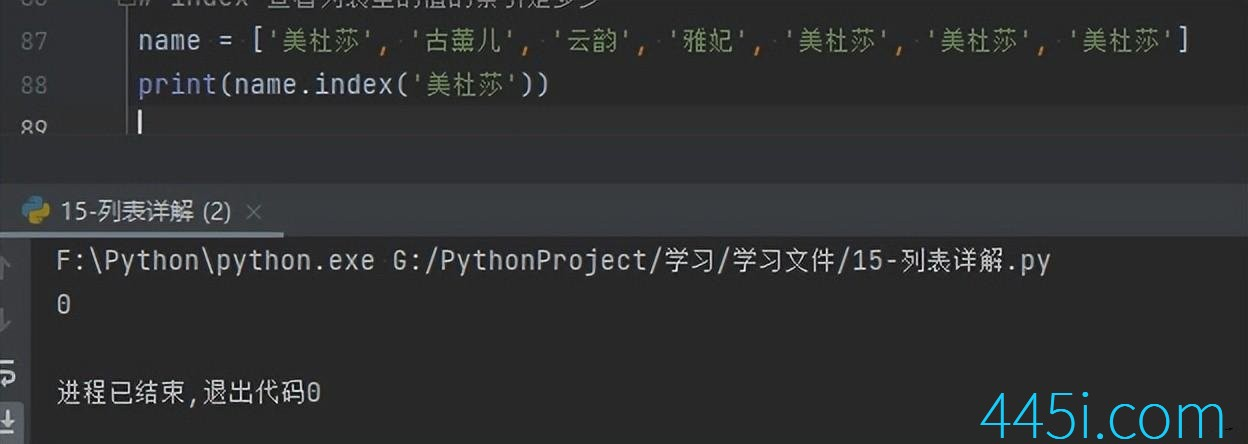### 觉得文章有用就打赏一下文章作者

#### 支付宝扫一扫打赏#### 微信扫一扫打赏